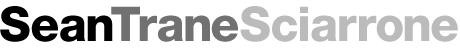This is a style guide to illustrate markdown syntax and the presentation styles applied to the output.

# Heading 1


## Text styling

- *italic*
- **bold**
- ~~strikethrough~~
- _underline_
- *_italic and underlined_*
- **_bold and underlined_**
- ==highlight==
- code()
- superscript^2y
- complex-superscript^(2y + 3z)

• italic
• bold
• strikethrough
• underline
• _italic and underlined_
• bold and underlined
• ==highlight==
• code()
• superscript^2y
• complex-superscript^(2y + 3z)

## Lists

* Thing one with an asterisk
+ Thing two with a plus
- Thing three with a minus

• Thing one with an asterisk
• Thing two with a plus
• Thing three with a minus
1. The first order of business
2. The second order of business
* The first part of the 2nd order
* The second part of the 2nd order
3. The third order of business

1. The first order of business
2. The second order of business
• The first part of the 2nd order
• The second part of the 2nd order
3. The third order of business

[This is an external link to Google](https://www.google.com/)
[This is a relative link to elsewhere in the site](/projects/)
[This is a link with a custom title](https://github.com/ "GitHub")


This is a relative link to elsewhere in the site

This is a link with a custom title

## Named Anchors

[Anchor Link](#anchor)

Anchor Placement <a id="anchor"></a>


Anchor Placement

## Images

Images work like links, but with a prefaced !. This supports SVG as well PNG, JPG, etc…

![](/images/seantrane.png)HTML can also be used, so sizing adjustments can be done with:

<img style="width:100px;" src="/images/seantrane.png"/>## Code

Inline monospaced-font can be made by surrounding text with back-ticks.


A bit of inline monospaced font can be made by surrounding text with back-ticks.

Larger code blocks can be made by surrounding the block with three back-ticks , and code can be highlighted by specifying language after the back-ticks, like so:

javascript
var s = "JavaScript syntax highlighting";


ruby
s = "Ruby syntax highlighting";
puts s


var s = "JavaScript syntax highlighting";

s = "Ruby syntax highlighting";
puts s


## Tables

column A | column B | column C | column D
--------:| -------- | -------- | --------
1A       | 1B       | 1C       | 1D
2A       | 2B       | 2C       | 2D
3A       | 3B       | 3C       | 3D

column A column B column C column D
1A 1B 1C 1D
2A 2B 2C 2D
3A 3B 3C 3D

## Block-quotes

> The data we have is not the data we want, and the data we need is not the data we have.


The data we have is not the data we want, and the data we need is not the data we have.

> Block-quote
>> Nested Block-quote


Block-quote

Nested Block-quote

## Horizontal rule

***
---
___


## Line breaks

Here's a line for us to start with.

This line is separated by two newlines, so it will be a separate paragraph.

This line is also a separate paragraph, though...
Adding a single line break does not create a new paragraph.


This line is separated by two newlines, so it will be a separate paragraph.

This line is also a separate paragraph, though… Adding a single line break does not create a new paragraph.

Use two trailing spaces
on the right
to create line-break tags


Use two trailing spaces on the right to create line-break tags

## Equations

Equations are rendered using KaTeX and take the follow LaTeX syntax. MathJax is a JavaScript display engine for mathematics that works in all browsers.

It is easy to use the MathJax library, it simply needs to be loaded on the site and pages where the equations must be processed.

As a standard precaution, equations in Markdown should be put inside HTML div block to prevent Markdown-processing from trying to parse the equation (and messing it up).

There are convenient ways, when using Jekyll, to activate MathJax only on pages that require it by using Jekyll’s Front Matter configuration blocks. A simple math: true can be used in front matter, and accessed in the template using a variable; {{ page.math }}.

Load MathJax Example (using Liquid conditional statement):

{% if page.math %}
<script src="https://cdn.mathjax.org/mathjax/latest/MathJax.js?config=TeX-AMS-MML_HTMLorMML"></script>
{% endif %}


Example equation in Markdown:

<div>
$$f(x) = \sum_{k=1}^{K}\alpha_{k}$$
</div>

$$f(x) = \sum_{k=1}^{K}\alpha_{k}$$

If MathJax has been loaded on this page, then the code above should be properly converted into an equation.

Inline-equations are too buggy with Markdown for this default approach to work properly. The following option attempts to solve that problem.

### Equations using Jekyll, Kramdown and Liquid

When using Jekyll and its default Kramdown parser, which has MathJax built into it, equations can be delimited using Liquid tags.

For Github Pages using Jekyll; there can be issues with LaTeX math in markdown files. The recommended solution is to use kramdown parser, with input: GFM configuration option (for GitHub-flavored Markdown).

Inline equations:

{% eqinline \dot{x} = \sigma(y-x) %}


Displayed equations:

{% eq \left( \sum_{k=1}^n a_k b_k \right)^2 \leq \left( \sum_{k=1}^n a_k^2 \right) \left( \sum_{k=1}^n b_k^2 \right) %}
`

The Liquid code above will cause errors if Kramdown is not the active Markdown parser.